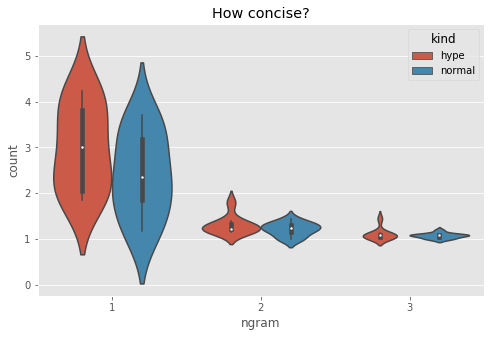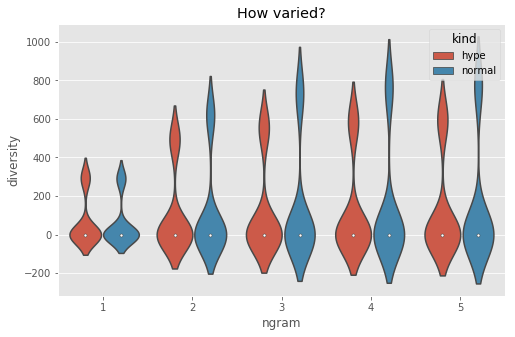/home /blog 30 Mar 2020 | Get ipynb

# Signal & Noise

``````How to learn when the topic is drowned out by hype
``````

This is the code accompanying the talk Signal&Noise

```# %pylab inline
plt.style.use("ggplot")

from collections import Counter, defaultdict
import pandas as pd
import seaborn as sns
import requests
from bs4 import BeautifulSoup
from urllib.parse import urlparse, parse_qs, urlencode
from newspaper import Article
import spacy

def search(q):
"""
We'll search google for the string provided and then return the content of the page as plain text.
"""
r = requests.get("https://www.google.com/search?" + urlencode({"q": q}))
soup = BeautifulSoup(r.text, "lxml")

links = [i for i in soup.find_all("a")]

parse_qs(urlparse(l).query)["q"] for l in links if l.startswith("/url?")
]

articles = []
try:
a.parse()
except:
pass
else:
if a.text.strip() != "":
articles.append(a.text)
return articles
```
```Populating the interactive namespace from numpy and matplotlib
```

Let's search for two topics. One that is clearly in the hype cycle and another that's the workhorse of computer science but is not being hyped about.

```ml = search("machine learning basics tutorial")
cpu = search("cpu scheduling basics tutorial")
```

Let's measure n grams on the articles returned by the search. We'll consider up to 6-grams

```N = 6

texts = [
[
i
for i in [
w.text.lower().strip() for w in nlp.tokenizer(t) if not w.is_punct
]
if i != ""
]
for t in texts
]
rows = []
for n in range(max_n):
grams = [list(zip(*[t[i:] for i in range(n)])) for t in texts]
for index, gr in enumerate(grams):
for key, val in Counter(gr).items():
rows.append([index, n, key, val])
df = pd.DataFrame(rows, columns=["doc", "ngram", "tpl", "count"])
return df

```

Of these two topics, which one has more words but fewer concepts? A larger number of 1 grams and higher 4-6 grams would mean that people are typing a lot of stuff. The articles require more explanation?

```mldf["kind"] = "hype"
cpudf["kind"] = "standard"
df = pd.DataFrame(
[
[count, n, kind]
for kind, df in [("hype", mldf), ("normal", cpudf)]
for n in range(4)
for count in df.loc[df.ngram == n].groupby("doc")["count"].mean()
]
)
df.columns = ["count", "ngram", "kind"]
plt.figure(figsize=(8, 5))
sns.violinplot(x="ngram", y="count", hue="kind", data=df)
plt.title("How concise?")
```
`Text(0.5,1,'How concise?')`Let's see how different each article is from the others?

```mldf["kind"] = "hype"
cpudf["kind"] = "standard"

def variety(df):
multisets = defaultdict(dict)
docids = set(df.doc.values)
for ngram in sorted(df.ngram.unique()):
part = df.loc[df.ngram == ngram]
for docid in docids:
part = part.loc[part.doc == docid]
for _, row in part.iterrows():
multisets[docid, ngram][row.tpl] = 1
rows = []
for ngram in df.ngram.unique():
for this in docids:
for other in docids - set([this]):
a = set(multisets[this, ngram])
b = set(multisets[other, ngram])
count = len(a - b) + len(b - a)
rows.append((ngram, count))
return pd.DataFrame(rows, columns=["ngram", "diversity"])

mldfv = variety(mldf)
mldfv["kind"] = "hype"
cpudfv = variety(cpudf)
cpudfv["kind"] = "normal"
plt.figure(figsize=(8, 5))
sns.violinplot(x="ngram", y="diversity", data=pd.concat([mldfv, cpudfv]), hue="kind")
plt.title("How varied?")
```
`Text(0.5,1,'How varied?')`